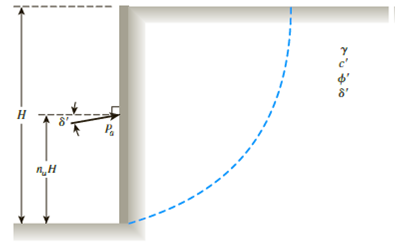Chapter 14, Problem 14.12PPrinciples of Geotechnical Enginee...

9th Edition
Braja M. Das + 1 other
ISBN: 9781305970939

Solutions

Chapter
SectionPrinciples of Geotechnical Enginee...

9th Edition
Braja M. Das + 1 other
ISBN: 9781305970939
Textbook Problem

A braced wall is shown in Figure 14.20. Given: H = 7 m, naH = 2.8 m, ϕ ′ = 30 ° , δ ′ = 20 ° , γ = 18 kN/m3, and c′ = 0. Determine the active thrust, Pa, on the wall using the general wedge theory.Figure 14.20

To determine

Find the active thrust Pa on the wall using the general wedge theory.

Explanation

Given information:

The height (H) of the retaining wall is 7.0 m.

The soil friction angle ϕ is 30°.

The angle of wall friction δ is 20°.

The unit weight γ of the granular backfill is 18kN/m3.

Calculation:

Determine the triangle pressure distribution na using the relation.

H=naH

Here, naH is the point of application of the active thrust.

Substitute 7.0 m for H and 2.8 m for naH.

7.0=na(2.8)na=2.87.0na=0.40

Calculate the active thrust Pa on the wall using the general wedge theory.

Pa0.5γH2=na

Refer Table 14.8 “Pa/0.5γH2 against ϕ, δ, and na(c=0) for braced cuts” in the text book

Still sussing out bartleby?

Check out a sample textbook solution.

See a sample solution

The Solution to Your Study Problems

Bartleby provides explanations to thousands of textbook problems written by our experts, many with advanced degrees!

Get Started

List three key points in caring for precision measuring tool.

Precision Machining Technology (MindTap Course List)

What are the basic components of a belt alternator starter hybrid system?

Automotive Technology: A Systems Approach (MindTap Course List)

How might small business and home users benefit from a P2P network? (457)

Enhanced Discovering Computers 2017 (Shelly Cashman Series) (MindTap Course List)

What types of protection are available to protect the ears during welding?

Welding: Principles and Applications (MindTap Course List)